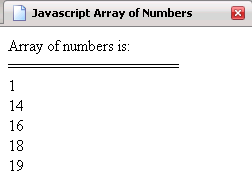Tutorials

# JavaScript array of numbers

In this tutorial we want to describe you a code that helps you in understanding 'Java Script array of numbers'.

In this tutorial we want to describe you a code that helps you in understanding 'Java Script array of numbers'.

# JavaScript array of numbers

In this tutorial we want to describe you a code that helps you in understanding 'Java Script array of numbers'. For this we use  Java Script language  as scripting language. We declare an array variable that is used to store array object. An array component  is accessed by an array expression that consists of an expression whose value is an array reference indicated by subscripts indexing expression enclosed by [].The for loop execute the script till the variable i is less than the length of an array.The document. write print the list of arrays of numbers.

Arrayofnumbers.html

 ```      Javascript Array of Numbers            var array=new Array();   array=1;   array=14;   array=16;   array=18;   array=19;   document.write("Array of numbers is: "+"
");   document.write("==================="+"
");   for(var i=0;i");   }       ```

Output of the program